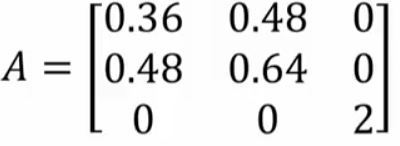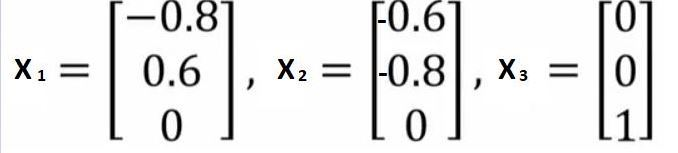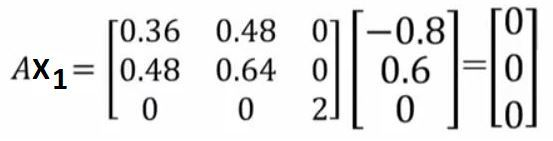# Connection Between Eigenvectors and Nullspace

Prerequisites:

Some important points about eigenvalues and eigenvectors:

• Eigenvalues can be complex numbers even for real matrices.
• When eigenvalues become complex, eigenvectors also become complex.
• If the matrix is symmetric (e.g A = AT), then the eigenvalues are always real.
• As a result, eigenvectors of symmetric matrices are also real.
• There will always be n linearly independent eigenvectors for symmetric matrices.

Now, let’s discuss the connection between eigenvectors and nullspace.

AX = λX

Now let me ask you a question. What happens when lambda is 0? That is one of the eigenvalues becomes 0.
So, when one of the eigenvalues becomes 0, then we have this equation which is given by

AX = 0 —(equation 1)

AB = 0 —(equation 2)

So you notice that equation 1 and equation 2 form are the same.

So, that basically means that X which is an eigenvector corresponding to eigenvalue, lambda equals to 0, is a null space vector, because it is just of the form that we have noticed here. So, we could say, the eigenvectors corresponding to zero eigenvalues are in the null space of the original matrix A. Conversely, if the eigenvalue corresponding to an eigenvector is not 0, then that eigenvector can not be in the null space of A. So, these are important results that we need to know.
So, this is how eigenvectors are connected to nullspace.

Example:
Consider the following A matrixNotice that this is a symmetric matrix hence the eigenvalues are always real as I told before in the important points section.
The eigenvalues for this matrix are

λ = (0, 1, 2)

The eigenvectors corresponding to these eigenvalues areCode: Python code to calculate eigenvalue and eigenvector

 `# Python program to illustrate ` `# connection between eigenvectors and nullspace ` ` `  `# Importing required libraries ` `import` `numpy as np ` `from` `numpy ``import` `linalg ` ` `  `# Taking A matrix ` `A ``=` `np.array([ ` `    ``[``0.36``, ``0.48``, ``0``], ` `    ``[``0.48``, ``0.64``, ``0``], ` `    ``[``0``, ``0``, ``2``] ` `]) ` ` `  `# Calculating eigenvalues and eigenvectors ` `eigValues, eigVectors ``=` `linalg.eig(A) ` ` `  `# Printing those values ` `print``(``"Eigenvalue are :"``, eigValues) ` `print``(``"Eigenvectors are :"``, eigVectors) ` ` `  `# Taking eigenvector 1 ` `eigVector1 ``=` `np.array([ ` `    ``[``-``0.8``], ` `    ``[``0.6``], ` `    ``[``0``] ` `]) ` ` `  `# Matrix multiplication between A and eigenvector1 ` `result ``=` `np.dot(A, eigVector1) ` `# Print the result ` `print``(result) ` ` `  `# This code is contributed by Amiya Rout `

```Output:
Eigenvalue are : [0. 1. 2.]
Eigenvectors are :
[[-0.8 -0.6  0. ]
[ 0.6 -0.8  0. ]
[ 0.   0.   1. ]]
[[0.]
[0.]
[0.]]
```

So we have noticed from our discussion before that if X1 is an eigenvector corresponding to lambda equal to 0, then this is going to be in the null space of this matrix A. Let’s verify it by multiplying A with X1. We check thatYou can quite easily see that when you do this computation, you will get this (0, 0, 0), which basically shows that this is the eigenvector corresponding to zero eigenvalue.

Don’t stop now and take your learning to the next level. Learn all the important concepts of Data Structures and Algorithms with the help of the most trusted course: DSA Self Paced. Become industry ready at a student-friendly price.

My Personal Notes arrow_drop_upCheck out this Author's contributed articles.

If you like GeeksforGeeks and would like to contribute, you can also write an article using contribute.geeksforgeeks.org or mail your article to contribute@geeksforgeeks.org. See your article appearing on the GeeksforGeeks main page and help other Geeks.

Please Improve this article if you find anything incorrect by clicking on the "Improve Article" button below.

Article Tags :

Be the First to upvote.

Please write to us at contribute@geeksforgeeks.org to report any issue with the above content.